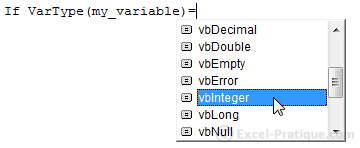# VBA Course: Conditions (continued)

## A conditional based on type

IsNumeric (this is the function we used on the last page) returns TRUE if the value is numerical and FALSE if this is not the case:

`Copy`If IsNumeric(Range("A1")) = True Then 'IF THE VALUE IS NUMERICAL ...``

The following code has the same effect as the version above (we don't have to include the = True because it is the default to ask whether a condition is true):

`Copy`If IsNumeric(Range("A1")) Then 'IF THE VALUE IS NUMERICAL ...``

If what we wanted to do was to test whether the value was NOT numerical, we could do this in either of the following two ways:

`Copy`If IsNumeric(Range("A1")) = False Then 'IF THE VALUE IS NOT NUMERICAL...``
`Copy`If Not IsNumeric(Range("A1")) Then 'IF THE VALUE IS NOT NUMERICAL ...``

Here are some examples of other functions similar to IsNumeric:

`Copy`If IsDate(Range("A1")) Then 'IF THE VALUE IS A DATE ...``
`Copy`If IsEmpty(Range("A1")) Then 'IF EMPTY...``
`Copy`If var_object Is Nothing Then 'IF OBJECT IS NOT SET ...``

## A conditional based on a variable's type

To execute commands based on the type of a variable (Variant), we will need to use the VarType function.

The list of types will appear once we have entered the = operator:`Copy`If VarType(my_variable) = vbInteger Then 'IF my_variable if is of type Integer ...``

Values of constants:

ConstantValue
vbEmpty0
vbNull1
vbInteger2
vbLong3
vbSingle4
vbDouble5
vbCurrency6
vbDate7
vbString8
vbObject9
vbError10
```Copy```If VarType(my_variable) = vbInteger Then 'IF my_variable is of type Integer ...
'Identical to:
If VarType(my_variable) = 2 Then 'IF my_variable is of type Integer ...``````

## A conditional based on comparing two character strings

Up to this point, we have seen this:

```Copy```my_variable = "Example 12345"

If my_variable = "Example 12345" Then ' => TRUE``````

In this case, the two strings are identical, it's nothing out of the ordinary ...

But if we want to test whether the variable contains the value "12345" without taking any of the other characters into account, we would have to use the Like command and a * operator before and after the search value.

The * operator stands in for: any character or multiple characters:

```Copy```my_variable = "Example 12345"

If my_variable Like "*12345*" Then ' => TRUE``````

The # operator can stand in for any numerical character from 0 to 9:

```Copy```my_variable = "Example 12345"

If my_variable Like "Example 12###" Then ' => TRUE``````

The ? operator can stand in for any single character:

```Copy```my_variable = "Example 12345"

If my_variable Like "?xample?1234?" Then ' => TRUE``````

We can also use a particular character or range of characters in the same way:

• [abc] stands in for any one of the following characters: a b c
• [a-g] stands in for any one of the following characters: a b c d e f g
•  stands in for any one of the following characters : 3 6 9
• [2-5] stands in for any one of the following characters: 2 3 4 5
• [?*#] stands in for any one of the following characters: ? * #
```Copy```my_variable = "Example 12345"

If my_variable Like "[DEF]xample 1234[4-7]" Then ' => TRUE``````

An ! added after the [ will stand in for any character not included within the bracketed expression:

```Copy```my_variable = "Example 12345"

If my_variable Like "[!GHIJ]xample 1234[!6-9]" Then ' => TRUE``````
An uppercase character is not equivalent to the same character in lowercase in this context. If you don't want to make distinctions between upper and lowercase characters, put an Option Compare Text command at the beginning of the module.